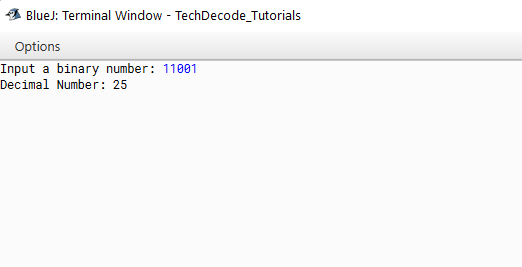# How to Convert Binary to Decimal in Java

Today let us know about How to Convert Binary to decimal in Java but before all let us know about Binary and Decimal numbers a bit.  A binary number system is one of the four types of the number system. In computer applications, where binary numbers are represented by only two symbols or digits, 0(zero) and 1(one). The binary numbers here are expressed in the base-2 numeral system. For example, (101) is a binary number.

Decimal is a term that describes the base-10 number system, probably the most commonly used number system. The decimal number system consists of ten single-digit numbers: 0, 1, 2, 3, 4, 5, 6, 7, 8, and 9. The number after 9 is 10.

## What’s the approach?

• Import the `java.util` package into the class.

• Create the `Main` Function

• Declare a `Scanner` Class to Accept input from the user.

• Initialize `4 long` variables

• Check if the `input` from the user is not `equal` to zero

• Extract the last digit by using `modulus`.

• The decimal number is calculated as per the formula.

• Repeat steps 6 and 7 until the number equals zero. After each iteration number is changed to` number/10.`

• Print the `decimal` number.

Also Read:- How to convert Int to Long in Java.

### Java Program to Convert Binary to Decimal

```/*

* TechDecode Tutorials

*

* How to Convert Binary to Decimal

*

*/

import java.util.*;

class Binary_to_Decimal

{

public static void main(String args[])

{

//Declaring Scanner Class

Scanner sc=new Scanner (System.in);

//Initializing varible

long bin;

long dec = 0;

long j = 1;

long remainder;

System.out.print("Input a binary number: ");

//Accpeting users input

bin = sc.nextLong();

//Converting to Decimal

while (bin != 0)

{

remainder = bin % 10;

dec = dec + remainder * j;

j = j * 2;

bin = bin / 10;

}

//Printing

System.out.println("Decimal Number: " + dec);

}

}```

Output:-